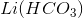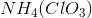# AP Chemistry : Solubility

## Example Questions

### Example Question #81 : Solutions

What is the formula for the dissociation of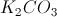?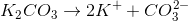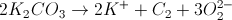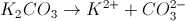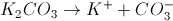Explanation:is composed of two types of ions,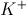and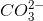. When it dissociates, it forms: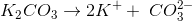### Example Question #82 : Solutions

Consider the following balanced equation for the solubility of barium hydroxide in an aqueous solution.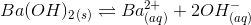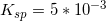What is the equilibrium expression for the balanced reaction?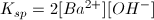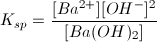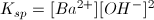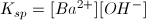Explanation:

When writing the equilibrium expression for an insoluble salt, remember that pure solids and liquids are not included in the expression. Also, the coefficients for the compounds in the balanced reaction become the exponents for the compounds in the equilibrium expression.

Given a generalized chemical reaction, we can determine the equilibrium constant expression.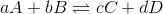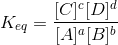In our reaction, the reactant is a pure solid and is not included in the equilibrium calculation.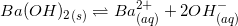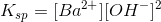### Example Question #83 : Solutions

Out of the compounds shown, which one is least soluble in water?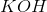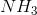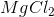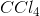Explanation:

This question is asking us to identify a compound that is the least soluble in the solvent water. To be able to answer this question, it's important to understand that, as a general rule of thumb, like dissolves like. In other words, since water is a polar molecule, the compounds that will best dissolve in water are those that are themselves either polar or carry a net charge.is expected to be soluble because it is polarized. With a lone electron pair on the nitrogen atom and the large electronegativity difference between the nitrogen and each of the three hydrogen atoms, ammonia will easily dissolve within water.

Likewise,is expected to dissolve in water because it can ionize. Once dissolved in water,can dissociate to become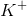and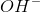. And since each of these molecules carry a charge and are thus very stabilized within the polar environment that water provides, the dissociation process is extremely favorable.

For much the same reasons,is also soluble in water. It can dissociate into one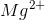and two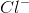, making it dissolve readily in water.

But what about? The difference in electronegativity between carbon and chlorine is high, so the compound is expected to be polar, right? Well, not exactly. There is a very important distinction to be made here. Just because a bond within a molecule is polar doesn't mean that the molecule itself is polar. Sure, the bond between carbon and chlorine is indeed polar. But remember, we also need to take the shape of the molecule into account.is a tetrahedral molecule. As a result, each of the chlorine atoms point away from the central carbon atom in the shape of a tetrahedron. Because of this, all of the individual carbon-chlorine dipoles (from each bond) cancels each other out. As a result of this, the net dipole moment on this compound becomes zero, and thus has no polarity. And since the polarity on this compound is zero, it would not be expected to dissolve very well in water.

### Example Question #84 : Solutions

Consider the solubility of the compound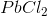. If the solubility of this compound is represented by, then which of the following is equal to the solubility product constant for?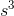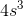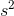Explanation:

In this question, we're given the solubility of a given compound, and we're asked to determine the solubility product constant of that compound.

When doing equilibrium problems that involve solubility, it's useful to construct "ICE" tables, which stands for initial, change, and equilibrium. What this means is that initial concentrations are written in the first row, then the change in concentration in the next row, and then these are summed together in the last equilibrium row.

To begin, we start by writing the equilibrium expression for the dissociation of this compound.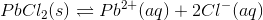Next, we construct an ICE table, as shown in the picture below.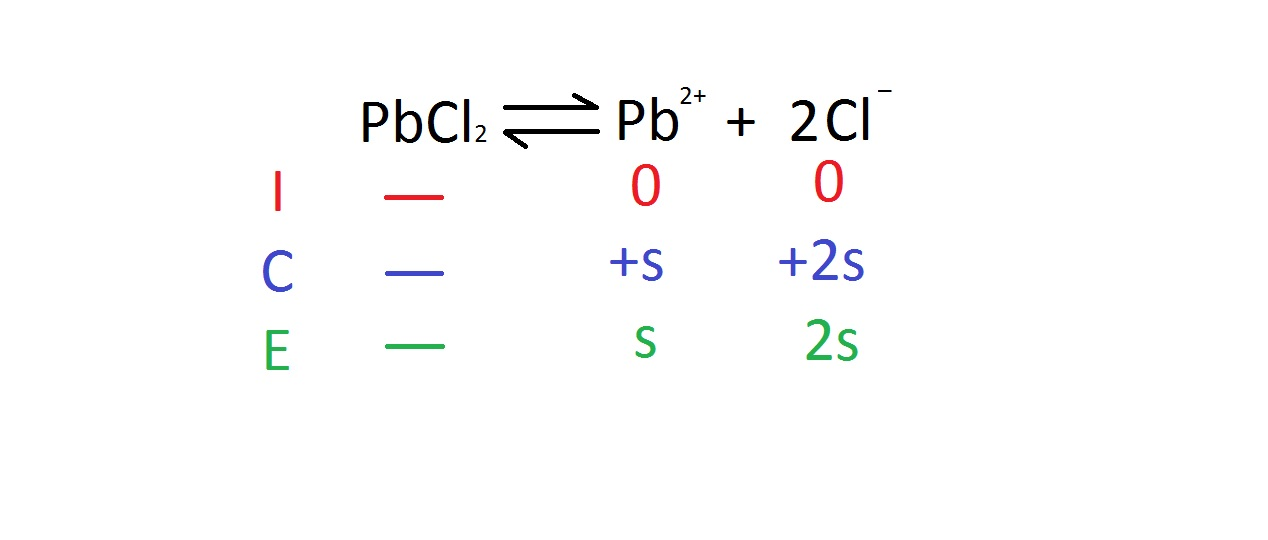Notice that we added onefor lead, butfor the chloride. This is because one lead is produced, but two chloride is produced as shown in the reaction. Also, notice that we don't include any concentration for the solid.

The next step is to write the expression for the equilibrium constant. Remember that solids don't appear in the expression.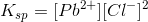The next step is to plug in the values from the ICE table to this expression.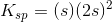Finally, we just need to simplify the expression into the following.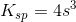### Example Question #85 : Solutions

Which of the following will be the precipitate in the following reaction?

2KCl + Ca(OH)2 → 2KOH + CaCl2

CaCl2

Ca

No precipitate forms

Ca(OH)2

KOH

No precipitate forms

Explanation:

Though the solubility of calcium hydroxide, Ca(OH)2, is fairly low, it is a reactant and will not form a precipitate. The solid calcium hydroxide will be added to an aqueous solution of potassium chloride, KCl. During the reaction, the calcium hydroxide will transition to potassium hydroxide (KOH) and calcium chloride (CaCl2), both of which are completely soluble. At the end of the reaction, no precipitate will be observed.

### Example Question #86 : Solutions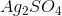is dissolved in water. Which of the following salts will result in a precipitate if added to the silver sulfate solution?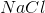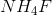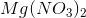Explanation:

This question requires an understanding of solubility guidelines and which ion combinations will result in precipitates. Virtually all ionic compounds containing ammonium, sodium, and nitrate will be soluble in water. Most ionic compounds containing chlorine are soluble, with the exceptions of silver, mercury, and lead cations.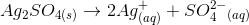If sodium chloride dissolves in this solution, the silver cations and chlorine anions will combine and result in a white, crystalline silver chloride precipitate.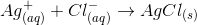### Example Question #87 : Solutions

Which of the following compounds is not soluble in water?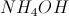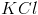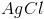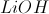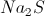Explanation:

The key to solubility is memorizing the solubility rules. We know that all compounds of alkali metals are soluble. These include potassium, lithium, and sodium, eliminating three of the answers. We also know that all chloride salts are soluble, except those of silver, lead, and mercury. Silver chloride is, thus, not soluble.

All hydroxide compounds are generally insoluble, but alkali metals and ammonium salts will make them soluble. Alkaline earth metals make hydroxides slightly soluble.

### Example Question #88 : Solutions

Which of these WILL be soluble in water?

CsCl

Ca2(PO4)3

AgCl

CuCl

FeCl2

CsCl

Explanation:

Solubility Rules: the only soluble ionic compound listed is CsCl; the rest are insoluble due to solubility rules

### Example Question #89 : Solutions

Which of the following compounds is insoluble in water?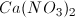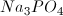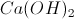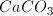Explanation:

Unless paired with an alkali metal, carbonate compounds are generally insoluble. Compounds that contain nitrate or an alkali metal will generally be soluble in water, and hydroxides are soluble when paired with heavier alkaline earth metals (such as calcium).

### Example Question #11 : Solubility

Which of the following will form a precipitate in solution?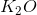None of these will form a precipitate